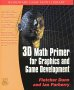# Maths - Using 4x4 matrix to represent rotation and translation

## Combined Rotation and Translation using 4x4 matrix.

A 4x4 matrix can represent all affine transformations (including translation, rotation around origin, reflection, glides, scale from origin contraction and expansion, shear, dilation, spiral similarities). On this page we are mostly interested in representing "proper" isometries, that is, translation with rotation.

We can combine two successive rotations about the origin by multiplying their matrices:

 r00 r01 r02 r10 r11 r12 r20 r21 r22
=
 ra00 ra01 ra02 ra10 ra11 ra12 ra20 ra21 ra22
*
 rb00 rb01 rb02 rb10 rb11 rb12 rb20 rb21 rb22

We can combine two successive translations by adding their vectors:

 t0 t1 t2
=
 ta0 ta1 ta2
+
 tb0 tb1 tb2

So how can we represent both rotation and translation in one transform matrix?

To do this we put the rotation matrix in columns and rows 0,1 and 2, we put the translation vector in the right column, the bottom row is 0,0,0,1.

 r00 r01 r02 t0 r10 r11 r12 t1 r20 r21 r22 t2 0 0 0 1

We can use this matrix to transform points or vectors. If we want to transform vectors then we represent it with the 4th row set to zero:

 x0 x1 x2 0

When multiplied by the above matrix the vector will be rotated only and not effected by the translation value. If however we want to transform points then we represent it with the 4th row set to one:

 x0 x1 x2 1

This will both rotate and transform the point.

To combine subsequent transforms we multiply the 4x4 matrices together. How is this multiplication of matrices equivalent to addition of the translation vectors?

One way to understand this is to realise that we are using projective geometry (see box on right).

An alternative way to show this is more of a brute force method; we can multiply two matrices representing pure translation and confirm that the translations get added:

 1 0 0 ta0 0 1 0 ta1 0 0 1 ta2 0 0 0 1
*
 1 0 0 tb0 0 1 0 tb1 0 0 1 tb2 0 0 0 1

The multiplication of two 4x4 matrices is:

 ma00*mb00 + ma01*mb10 + ma02*mb20 + ma03*mb30 ma00*mb01 + ma01*mb11 + ma02*mb21 + ma03*mb31 ma00*mb02 + ma01*mb12 + ma02*mb22 + ma03*mb32 ma00*mb03 + ma01*mb13 + ma02*mb23 + ma03*mb33 ma10*mb00 + ma11*mb10 + ma12*mb20 + ma13*mb30 ma10*mb01 + ma11*mb11 + ma12*mb21 + ma13*mb31 ma10*mb02 + ma11*mb12 + ma12*mb22 + ma13*mb32 ma10*mb03 + ma11*mb13 + ma12*mb23 + ma13*mb33 ma20*mb00 + ma21*mb10 + ma22*mb20 + ma23*mb30 ma20*mb01 + ma21*mb11 + ma22*mb21 + ma23*mb31 ma20*mb02 + ma21*mb12 + ma22*mb22 + ma23*mb32 ma20*mb03 + ma21*mb13 + ma22*mb23 + ma23*mb33 ma30*mb00 + ma31*mb10 + ma32*mb20 + ma33*mb30 ma30*mb01 + ma31*mb11 + ma32*mb21 + ma33*mb31 ma30*mb02 + ma31*mb12 + ma32*mb22 + ma33*mb32 ma30*mb03 + ma31*mb13 + ma32*mb23 + ma33*mb33

Substituting the values from the translation gives:

 1*1 + 0*0 + 0*0 + ta0*0 1*0 + 0*1 + 0*0 + ta0*0 1*0 + 0*0 + 0*1 + ta0*0 1*tb0 + 0*tb1 + 0*tb2 + ta0*1 0*1 + 1*0 + 0*0 + ta1*0 0*0 + 1*1 + 0*0 + ta1*0 0*0 + 1*0 + 0*1 + ta1*0 0*tb0 + 1*tb1 + 0*tb2 + ta1*1 0*1 + 0*0 + 1*0 + ta2*0 0*0 + 0*1 + 1*0 + ta2*0 0*0 + 0*0 + 1*1 + ta2*0 0*tb0 + 0*tb1 + 1*tb2 + ta2*1 0*1 + 0*0 + 0*0 + 1*0 0*0 + 0*1 + 0*0 + 1*0 0*0 + 0*0 + 0*1 + 1*0 0*tb0 + 0*tb1 + 0*tb2 + 1*1

Removing any terms containing 0 gives,

 1 0 0 tb0 + ta0 0 1 0 tb1 + ta1 0 0 1 tb2 + ta2 0 0 0 1

Which is the addition of the vectors as required?

## Inverting the Transformation

One way to reverse a trasformation is to invert the 4×4 matrix as described on this page. However the matrix carries a lot of redundant information, so if we want to speed up the code we can take advantage of this redundant information. If the matrix is normalised approriately then,

• to invert a pure rotation then we just take the transpose of the 3x3 part of the matrix.
• to invert a pure translation the we just negate the translation

so for a combined rotation and translation then we should be able to combine these but with some compensation for the rotation of the translation direction.

 metadata block see also: Correspondence about this page Book Shop - Further reading. Where I can, I have put links to Amazon for books that are relevant to the subject, click on the appropriate country flag to get more details of the book or to buy it from them.3D Math Primer - Aimed at complete beginners to vector and matrix algebra. Other Math Books